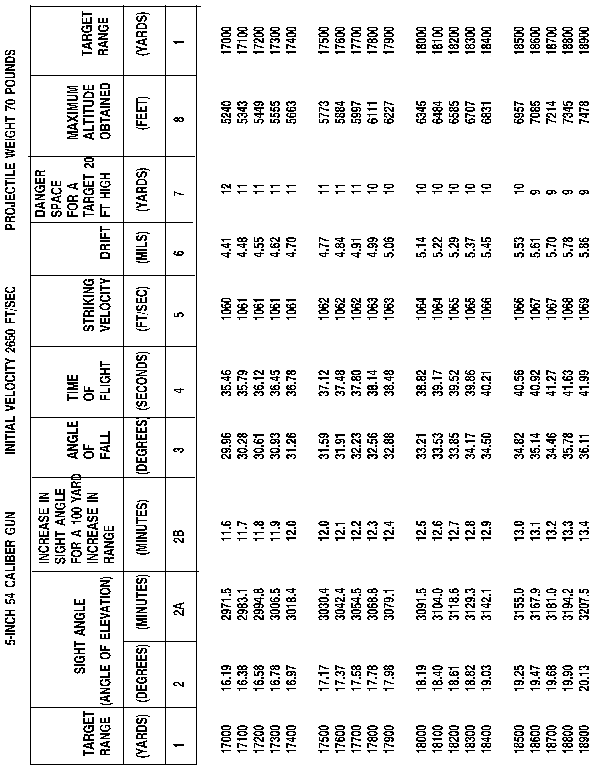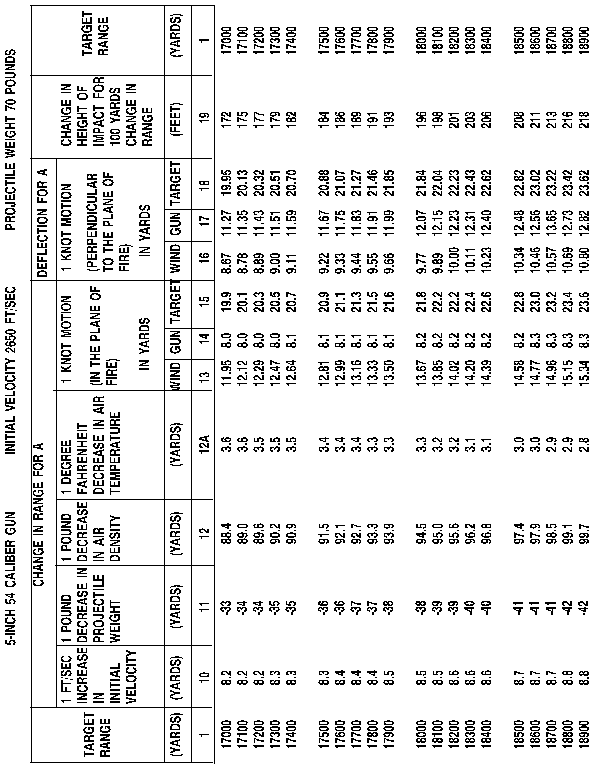####Gunnery Officer

INFORMATION SHEET

RANGE TABLES

Information Sheet Number: 1.8

INTRODUCTION

This lesson is an introduction to range table reading. It gives a column by column description of what information is contained and its applicability for 5"/54 MK 45 guns. This material will be used in the exterior ballistic corrections lecture.

REFERENCES

(a) SW323-AF-ORD-010 Range Tables for 5"/54 caliber guns

INFORMATION

A. THE RANGE TABLE IS DIVIDED INTO SIX SECTIONS

1. Section 1 - description of range table and an outline of standard conditions

a. Std initial velocity = 2650 FT/SEC

b. Std air temperature = 590 (F)

c. Std powder temperature = 900 (F)

d. Std projectile weight = 70 lbs

e. Std Atmosphere = 100%

f. Table 1-1 lists fuse combination applicability.

2. Section 2 - range table

3. Section 3 - initial ballistics

4. Section 4 - graphical trajectories

5. Section 5 - weapons dispersion table

6. Section 6 - exterior ballistic parametersB. DETAILED DESCRIPTION OF EACH SECTION

1. Section 1 - Establishes standard fuse/projectile combinations.

a. Lists equivalent density correction percentages

b. Lists projectile weights

2. Section 2 - range tables

a. Divided into two (2) groups

(1) Group 1 - Columns 1 - 8

(a) Describes trajectory characteristics under standard conditions.

(b) Refer to figure 1.8-1

(2) Group 2 - Columns 10 - 19

(a) Describes changes in range and deflection due to variations from standard conditions.

(b) Refer to figure 1.8-2

b. Column 1 - Target range

(1) Starts at 1000 yds, increments by 100 yds up to max range, after max range, decrements by 100 yards for reverse slope.

c. Column 2 - Sight angle elevation (Degrees)

(1) Gives gun angle elevation for corresponding ranges in degrees, tenths and hundredths under standard conditions.

d. Column 2A - Sight angle elevation (minutes)

(1) Same as column 2 - given in minutes.

e. Column 2B - Increase to sight angle elevation for every 100 yd range increase.

(1) Gives amount required (in minutes) to add 100 yd increase in present range.

f. Column 3 - Angle of fall

(1) Angle above horizontal that projectile will impact - in degrees, tenths and hundredths.

g. Column 4 - Time of flight

(1) From ignition of propellant to time of impact.

h. Column 5 - Striking velocity

(1) Velocity of projectile at impact (ft/sec).

i. Column 6 - Drift

(1) Distance to the right, in mils, projectile will impact for given range (column 1).

j. Column 7 - Danger space for a 20 ft target

(1) The distance in yards a target 20 ft tall can move and still be hit.

(2) For targets in excess of 20 ft, divide actual target height by 20, then multiply result by value in column 7.

k. Column 8 - Maximum ordinate

(1) Maximum altitude the projectile will attain during its flight (in feet).

l. Column 1 - Target range

(1) Repeated for user's convenience.

m. Column 1 - 8 are for standard conditions.

n. Columns 10 - 19, the next part of the lesson, are computed changes in range and deflection for deviations from standard conditions.

o. Column 10 - Change in range for every 1 ft/sec change in initial velocity

(1) Note that this is a change for an increase, for a one ft/sec decrease reverse the sign of the value in column 10.

(2) For more than a one (1) ft/sec deviation, multiply the value in column 10 by the total number of ft/sec deviation.

p. Column 11 - Change in range for a one (1) pound decrease in projectile weight.

(1) Note that this is a change for a decrease, for a 1 pound increase reverse the sign of the value in column 11.

(2) For more than 1 pound deviation, multiply the value in column 11 by the total number of pounds deviation.

q. Column 12 - Change in range for a 1% decrease in air density.

(1) Note that this is a change for a decrease, for a 1% increase reverse the sign of the value in column 12.

(2) For more than 1% deviation, multiply the value in column 12 by the total number of percent deviation.

r. Column 12A - change in range for a 1 degree (F) change in air temperature.

(1) Note that this is a change for a decrease, for a 1 degree (F) increase reverse the sign of the value in column 12A.

(2) For more than 1 degree (F) deviation, multiply the value in column 12A by the total number of degree (F) deviation.

(3) The functions defined in columns 13 through 18 are characteristics that are inherent to the gun fire control computer. These functions are presented for information only.

s. Column 13 - 14 - 15 change in range for 1 knot of motion perpendicular to the plane of fire of:

(1) Wind - column 13

(2) Gun - (own ship) column 14

(3) Target - column 15

t. Column 16 - 17 - 18 change in deflection for 1 knot of motion perpendicular to the plane of fire of:

(1) Wind - column 16

(2) Gun (own ship) - column 17

(3) Target - column 18

u. Column 19 - change in height of impact with a 100 yd change to target range.

(1) Note that this is a change in height based upon a 100 yd increase in target range.

(2) To determine the hitting space, divide target height by the value in column 19, then multiply by 100 yd.

3. Section 3 - Initial Ballistic Corrections (IBC)

a. One set of associated tables and figures is provided for each system configuration that utilizes the projectile.

b. It is recommended that the tables and figures that do not match your system configuration be removed from the manual and stored separately to preclude confusion.

c. The IBC computation sheet provides for variations from the following standards:

(1) Equivalent density

(2) Surface air temp

(3) Ballistic air density

(4) Ballistic wind speed and direction

(5) Projectile weight

(6) Powder temp

(7) Barrel wear

(8) Trunnion height - gun

(9) Earth curvature

(10) Range error

d. Terms used in this section

e. Computation sheet for MK 68 system utilizing MK 47 mods 5 & 7-11 computers

f. Directions for using the nomogram (surface observation)

g. Nomogram (surface density)

(1) Air temp vs. barometric pressure

h. Meteorological priority chart

(1) Determines the order of priority that ballistic wind and density sources should be used in the calculations.

i. Earth curvature chart

(1) Used to determine the range correction for earth curve

j. Velocity loss vs. Projectile Seating Distance (PSD).

(1) Positive values on this table indicate loss, negative values indicate gain in velocity.

(2) Powder coefficient chart

(a) IV Directly proportional to powder temp.

k. Gun trunnion height

4. Section 4 - Graphic trajectories

a. Illustrates the information contained in columns:

(1) Horizontal range

(2) Sight angle elevation

(3) Time of flight

(4) Maximum ordinate (altitude)

5. Section 5 - Weapons dispersion

a. Values represent the distance on either side of the mean point of impact that 67% of all rounds fired will fall for the corresponding range.

6. Section 6 - exterior ballistic parameters

C. RANGE TABLE SW323-AB-ORD-010

1. Same as SW323-AF-ORD-010 except it applies to reduced charge vice full service charge.

D. Other range tables 5"/54

1. Available for air targets

2. Available for illumination projectiles

3. Available for high frag rounds